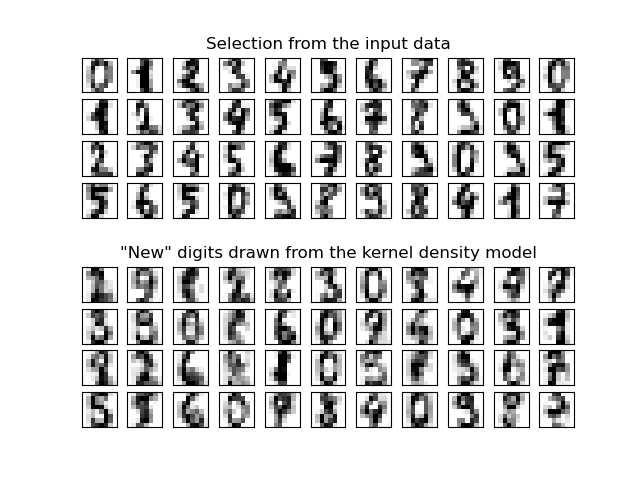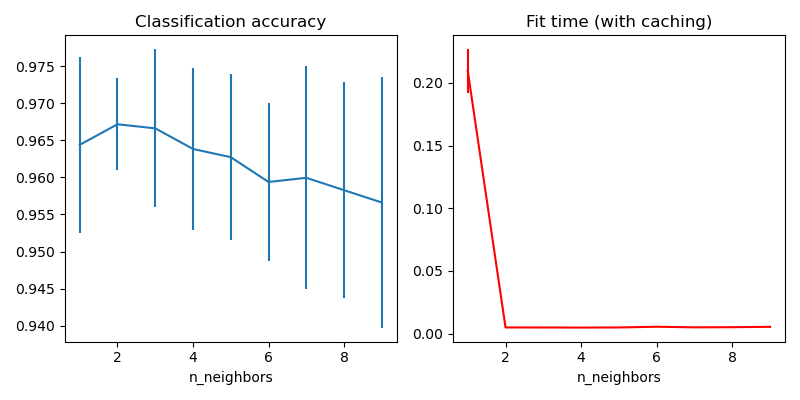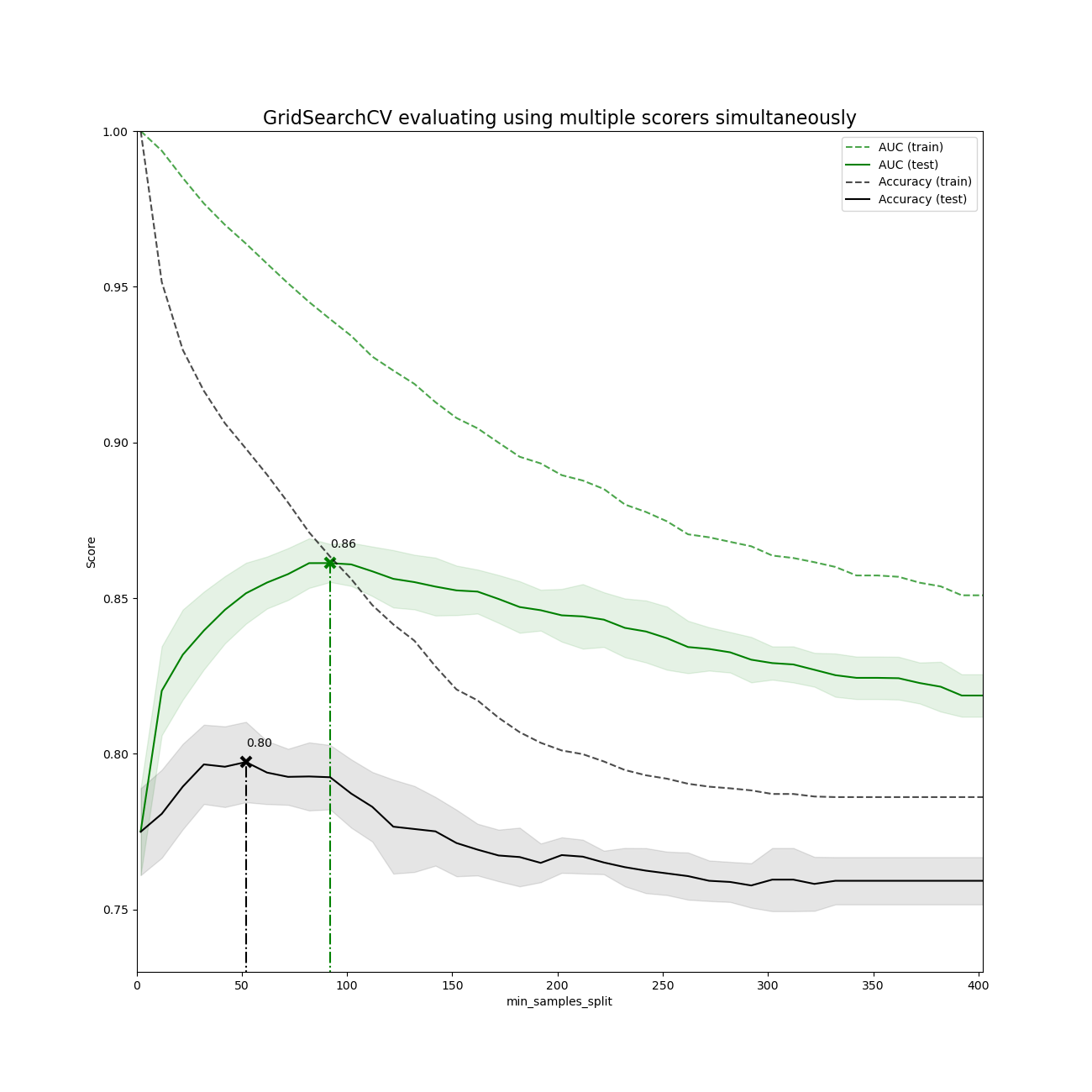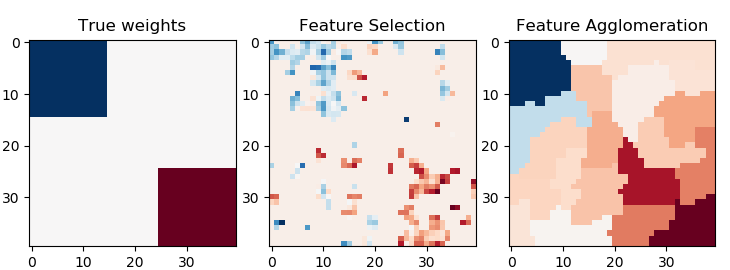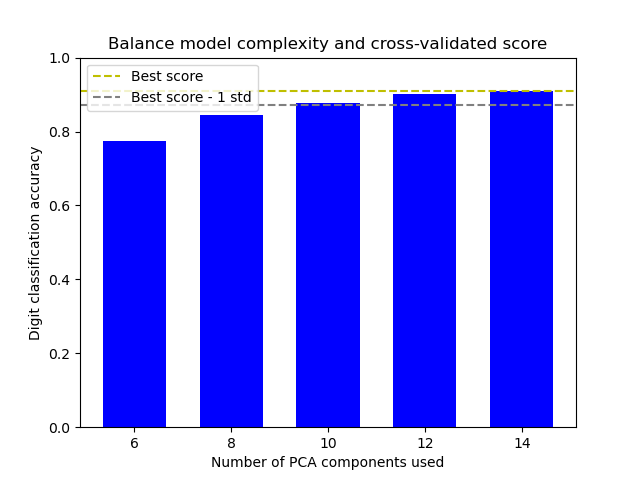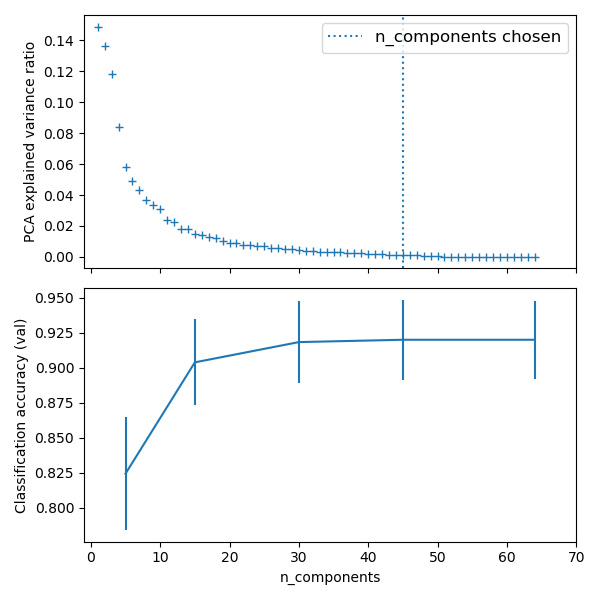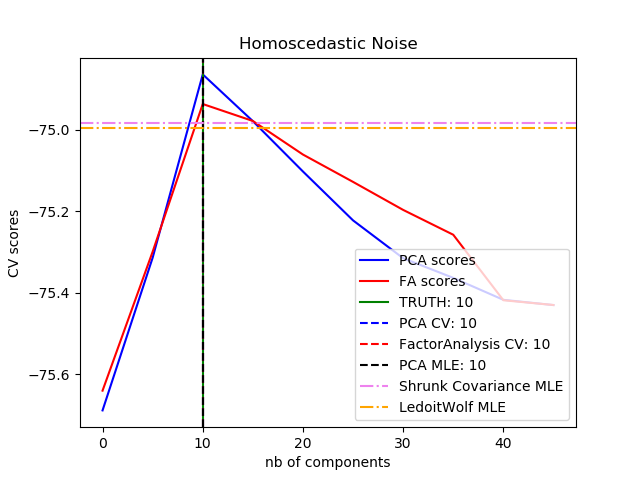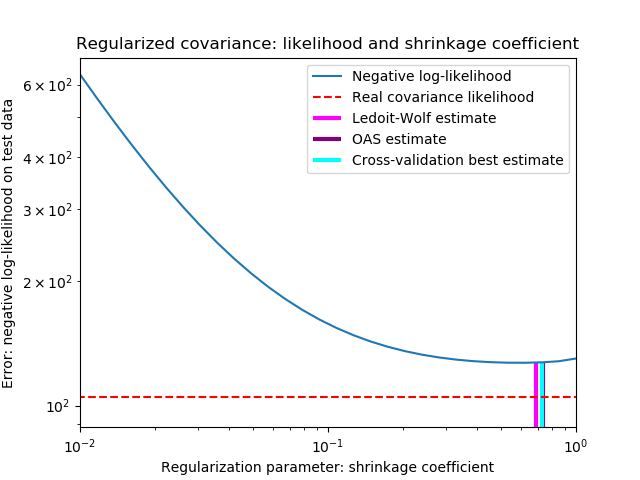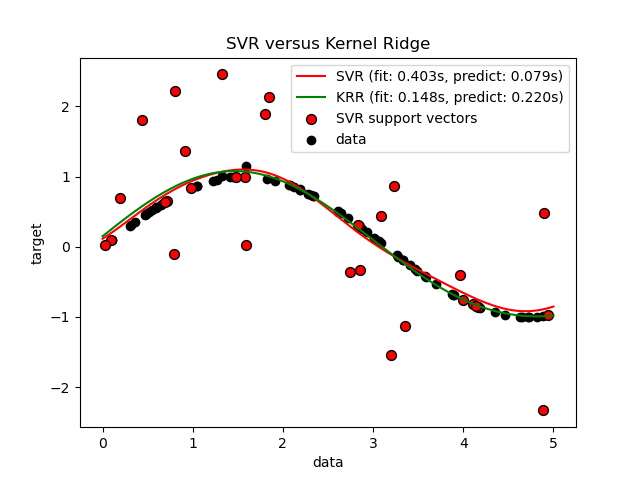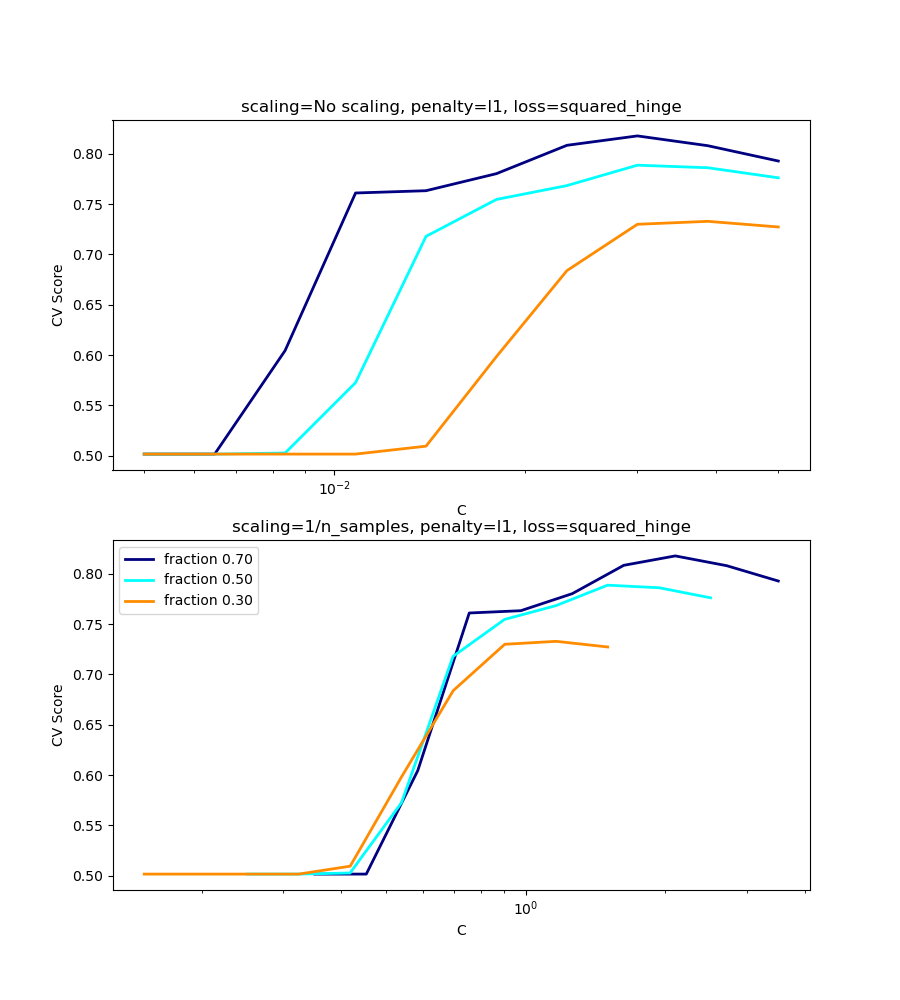# sklearn.model_selection.GridSearchCV¶

class sklearn.model_selection.GridSearchCV(estimator, param_grid, *, scoring=None, n_jobs=None, iid='deprecated', refit=True, cv=None, verbose=0, pre_dispatch='2*n_jobs', error_score=nan, return_train_score=False)

[源码]

GridSearchCV实现“拟合”和“得分”方法。如果在所使用的估计器中实现了“predict”，“predict_proba”，“decision_function”，“transform”和“inverse_transform” if the”，则还可以实现它们。

estimator estimator object

param_grid dict or list of dictionaries

scoring str, callable, list/tuple or dict, default=None

n_jobs int, default=None

v0.20版中的更改：n_jobs默认从1更改为None
pre_dispatch int, or str, default=n_jobs

- None,在这种情况下，所有CPU内核都将立即创建并产生，使它进行轻量级和快速运行的任务，以避免因按需生成作业而造成延迟
-int,给出所产生的总CPU内核数的确切数量
-str,根据n_jobs给出表达式，如'2*n_jobs'
iid bool, default=False

cv int, cross-validation generator or an iterable, default=None

-None,默认使用5折交叉验证
-integer,用于指定在(Stratified)KFold中的折叠次数
-CV splitter
-一个输出训练集和测试集切分为索引数组的迭代。

refit bool, str, or callable, default=True

verbose integer

error_score ‘raise’ or numeric, default=np.nan

return_train_score bool, default=False

cv_results_ dict of numpy (masked) ndarrays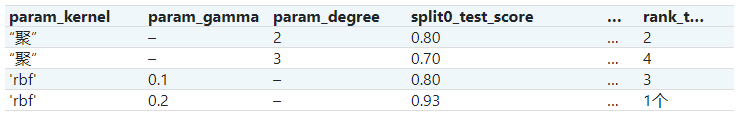用一个cv_results_字典表示：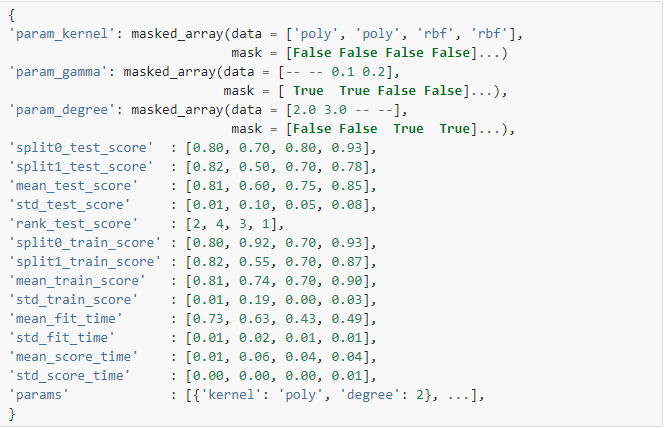注意
'params'键用于存储所有候选参数的参数设置字典列表。

mean_fit_timestd_fit_timemean_score_timestd_score_time的单位都是秒。

best_estimator_ estimator

best_score_ float
best_estimator的平均交叉验证得分

best_params_ dict

best_index_ search.cv_results_['params'][search.best_index_]的字典给出了最优模型的参数设置，该模型给出最高平均得分（search.best_score_）。

scorer_ function or a dict

n_splits_ int

refit_time_ float

0.20版中的新功能。

ParameterGrid 生成超参数网格的所有组合。

sklearn.model_selection.train_test_split 用于将数据切分为可用于拟合GridSearchCV实例的开发集和用于最终评估的验证集的实用程序功能。

sklearn.metrics.make_scorer 根据绩效指标或损失函数确定评分器。

>>> from sklearn import svm, datasets>>> from sklearn.model_selection import GridSearchCV>>> iris = datasets.load_iris()>>> parameters = {'kernel':('linear', 'rbf'), 'C':[1, 10]}>>> svc = svm.SVC()>>> clf = GridSearchCV(svc, parameters)>>> clf.fit(iris.data, iris.target)GridSearchCV(estimator=SVC(),             param_grid={'C': [1, 10], 'kernel': ('linear', 'rbf')})>>> sorted(clf.cv_results_.keys())['mean_fit_time', 'mean_score_time', 'mean_test_score',... 'param_C', 'param_kernel', 'params',... 'rank_test_score', 'split0_test_score',... 'split2_test_score', ... 'std_fit_time', 'std_score_time', 'std_test_score']

decision_function(self, X) 使用找到的最优参数在估计器上调用Decision_function。
fit(self, X[, y, groups]) 拟合所有参数组合。
get_params(self[, deep]) 获取此估计器的参数。
inverse_transform(self, Xt) 用找到的最优参数在估计器上调用inverse_transform。
predict(self, X) 使用找到的最优参数在估计器上调用预测。
predict_log_proba(self, X) 使用找到的最优参数在估计器上调用predict_log_proba。
predict_proba(self, X) 使用找到的最优参数在估计器上调用predict_proba。
score(self, X[, y]) 如果估计器已调整，则返回给定数据的分数。
set_params(self, **params) 设置此估计器的参数。
transform(self, X) 使用找到的最优参数在估计器上调用transform。
__init__（self，estimator，param_grid，*，scoring = None，n_jobs = None，iid ='deprecated'，refit = True，cv = None，verbose = 0，pre_dispatch ='2 * n_jobs'，error_score = nan，return_train_score = False ）

[源码]

decision_function(self, X)

[源码]

X indexable, length n_samples

fit（self，X，y = None，*，groups = None，** fit_params ）

[源码]

X array-like of shape (n_samples, n_features)

y array-like of shape (n_samples, n_output) or (n_samples,), default=None

groups array-like of shape (n_samples,), default=None

**fit_params dict of str -> object

get_params（self，deep = True ）

[源码]

deep bool, default=True

params mapping of string to any

inverse_transform(self, Xt)

[源码]

Xt indexable, length n_samples

predict(self, X)

[源码]

X indexable, length n_samples

predict_log_proba(self, X)

[源码]

X indexable, length n_samples

predict_proba(self, X)

[源码]

X indexable, length n_samples

score（self，X，y = None ）

[源码]

X array-like of shape (n_samples, n_features)

y array-like of shape (n_samples, n_output) or (n_samples,), default=None

score float
set_params(self, **params)

[源码]

**params dict

self object

transform(self, X)

[源码]

X indexable, length n_samples

## sklearn.model_selection.GridSearchCV使用示例¶